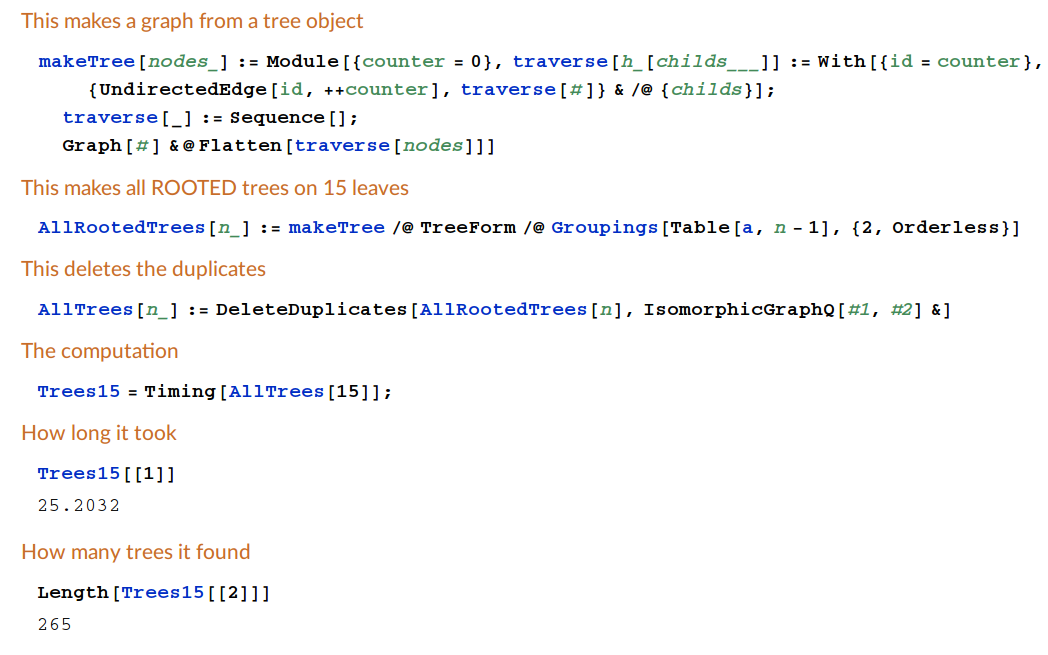# Problem 16

List all unlabeled trivalent phylogenetic trees with 15 leaves.

## Solution

Here is a solution in Mathematica:

This makes a graph from a tree object:

```makeTree[nodes_] :=
Module[{counter = 0},
traverse[h_[childs___]] := With[{id = counter}, {UndirectedEdge[id, ++counter],
traverse[#]} & /@ {childs}];
traverse[_] := Sequence[];
Graph[#] &@Flatten[traverse[nodes]]]
```

This makes all ROOTED trees on 15 leaves:

```AllRootedTrees[n_] := makeTree /@ TreeForm /@ Groupings[Table[a, n - 1], {2, Orderless}]
```

This deletes the duplicates:

```AllTrees[n_] := DeleteDuplicates[AllRootedTrees[n], IsomorphicGraphQ[#1, #2] &]
```

The computation:

```Trees15 = Timing[AllTrees];
```

How long it took:

```Trees15[]
```

How many trees it found:

```Length[Trees15[]]
```